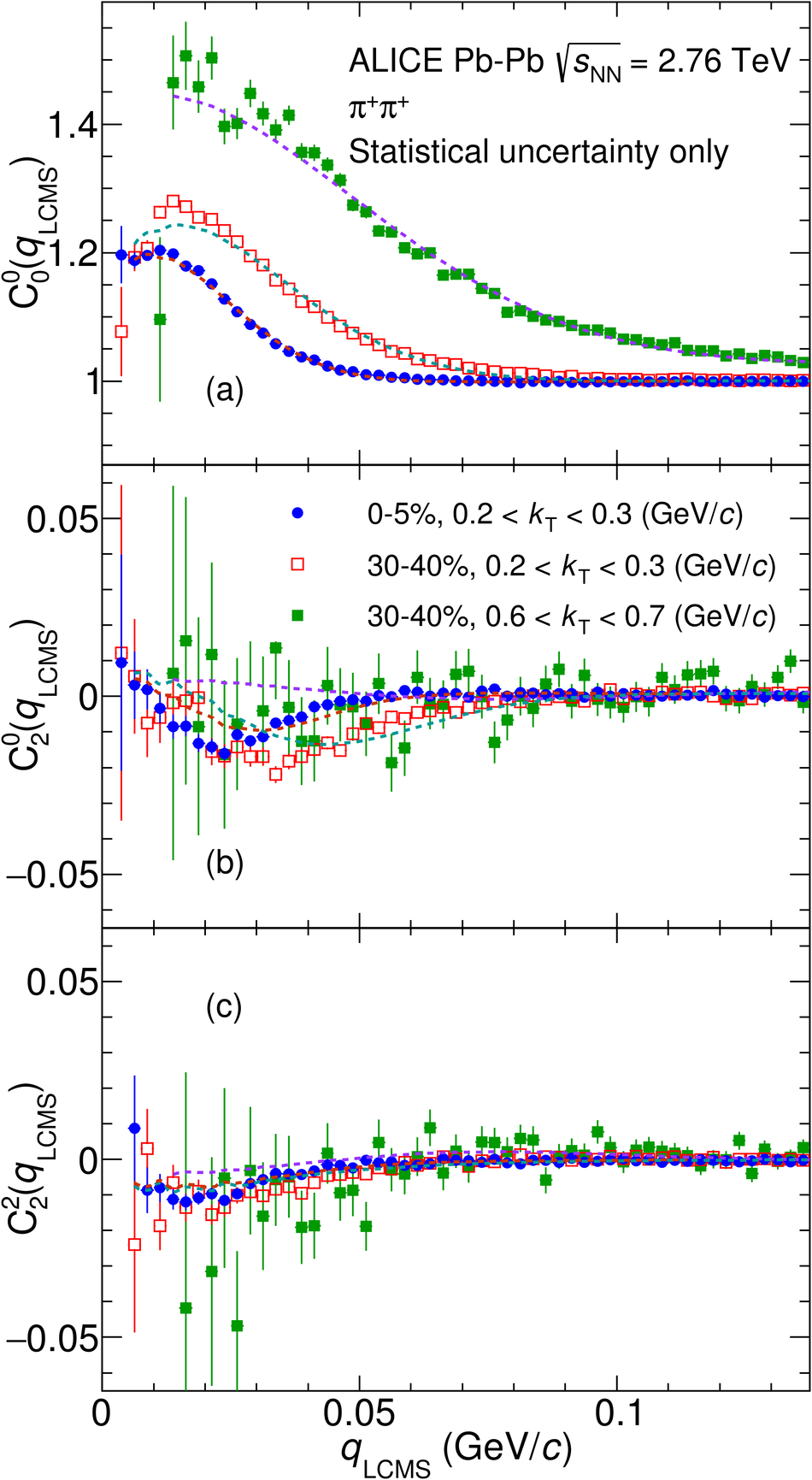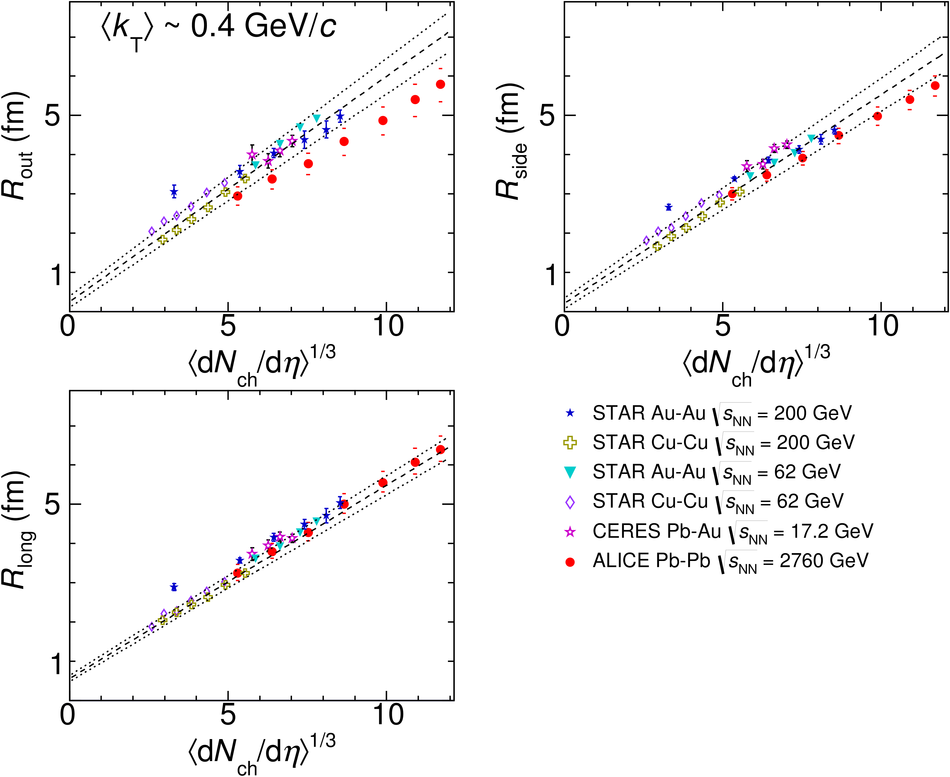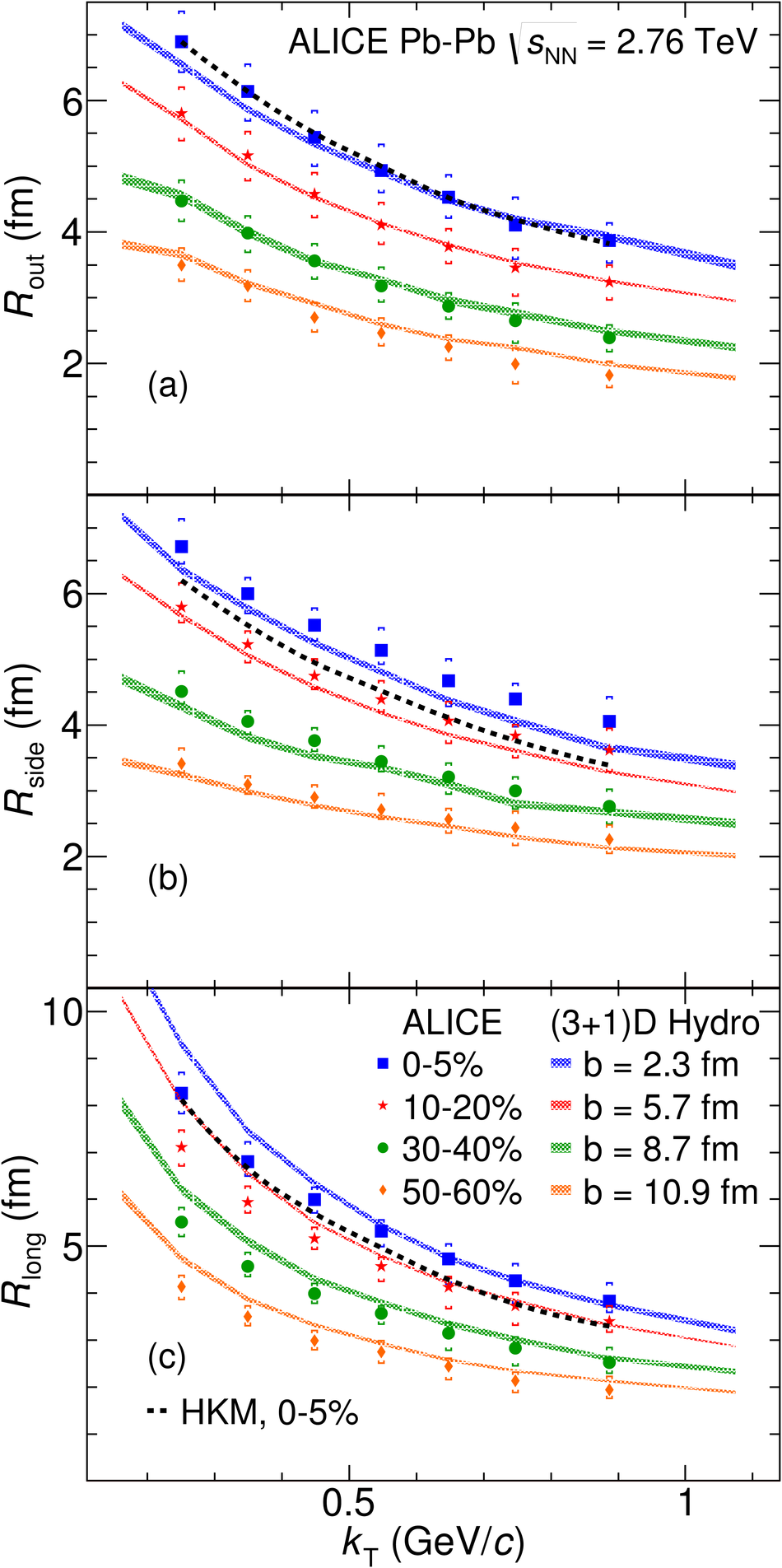# Centrality dependence of pion freeze-out radii in Pb-Pb collisions at $\sqrt{\mathbf{s_{NN}}}$=2.76 TeV

We report on the measurement of freeze-out radii for pairs of identical-charge pions measured in Pb--Pb collisions at $\sqrt{s_{\rm NN}}=2.76$ TeV as a function of collision centrality and the average transverse momentum of the pair $k_{\rm T}$. Three-dimensional sizes of the system (femtoscopic radii), as well as direction-averaged one-dimensional radii are extracted. The radii decrease with $k_{\rm T}$, following a power-law behavior. This is qualitatively consistent with expectations from a collectively expanding system, produced in hydrodynamic calculations. The radii also scale linearly with $\left<~ \mathrm{d}N_{\rm ch}/\mathrm{d}\eta \right>^{1/3}$. This behaviour is compared to world data on femtoscopic radii in heavy-ion collisions. While the dependence is qualitatively similar to results at smaller $\sqrt{s_{\rm NN}}$, a decrease in the $R_{\rm out}/R_{\rm side}$ ratio is seen, which is in qualitative agreement with specific predictions from hydrodynamic models. The results provide further evidence for the production of a collective, strongly coupled system in heavy-ion collisions at the LHC.

Figures

## Figure 1

 Projections of the Cartesian representation of the three-dimensional two-pion correlation functions along the $out$~(a), $side$~(b) and $long$~(c) axes. The centrality and pair momentum ranges for the three functions are given on the plot. In each case the other components are projected over $\pm$ 20 MeV/$c$ around 0 in the other $q$ directions for central collisions at low \kt and $\pm$ 33 MeV/$c$ in the other two cases. Lines represent the corresponding projections of the fit to the experimental correlation functions with formula from Eq.~\eqref{eq:cfitfun}.## Figure 2

 First three non-vanishing components of the SH representation of the two-pion correlation functions, $l=0,m=0$~in~(a), $l=2,m=0$~in~(b) and $l=2,m=2$~in~(c). The centrality and pair momentum ranges for the three functions are given on the plot. The lines show the corresponding SH components of the fit with formula from Eq.~\eqref{eq:cfitfun}.## Figure 3

 Femtoscopic radii as a function of pair transverse momentum \kt for seven centrality ranges. $R_{\rm out}$ is shown in (a), $R_{\rm side}$ in (b), $R_{\rm long}$ in (c) and $R_{\rm out}/R_{\rm side}$ in (d). The points for centralities 5--10\%, 10--20\%, 30--40\% and 40--50\% have been slightly shifted in the x direction for visibility. Statistical uncertainties are shown as black lines (usually smaller than symbol size), systematic uncertainties are shown as colored caps.## Figure 4

 The radii plotted as a function of \dnde ~for seven \kt ranges. $R_{\rm out}$ is plotted in(a), $R_{\rm side}$ in (b), and $R_{\rm long}$ in (c). Thedashed lines represent linear fits to each set of points for a givencomponent and \kt range. Points for some \kt ranges have beenslightly shifted in the $x$ direction for visibility. Statisticaluncertainties are shown as black lines (usually smaller than symbolsize), systematic uncertainties are shown as colored caps.## Figure 5

 The slope parameters of the linear fits shown in Fig.~\ref{fig:pbradmult}, as a function of pair \mt. Lines represent the power-law fits (see text for details). Errorbars represent the uncertainty of the parameter of the fits, in which the combined systematic and statistical uncertainties on the radii ware taken into account.## Figure 6

 One-dimensional femtoscopic radius $R_{\rm inv}$ as a function of pair transverse momentum \kt for seven centrality ranges. Points for 5--10\%, 20--30\%, and 40--50\% centrality have been slightly shifted in the $x$ direction for visibility. Statistical uncertainty is shown as black lines, systematic uncertainty is shown as colored caps.## Figure 7

 Comparison of femtoscopic radii, as a function of measured charged particle multiplicity, for a number of collision systems and collision energies~ Dashed lines show linear fits done to heavy-ion data, excluding ALICE (dotted lines show one sigma contour). Various experiments use differing \kt ranges, values shown on the plot are for the range for which the average \kt is closest to the selected value of $0.4$~GeV/$c$; in case of ALICE an average of two neighbouring ranges of \kt is shown. Systematic uncertainties are shown where available## Figure 8

 Comparison of the femtoscopic radii (\rout ~in (a), \rside~ in (b), and \rlong~ in (c)), as a function of pair transverse momentum, with the calculation from the Therminator and (3+1)D hydro model~, for four centralities (here identified by the value of the impact parameter $b$ used in the calculation) and with the HKM model~ for the central data. Closed symbols are experimental data (with statistical and systematic uncertainty), bands and dashed lines are the model calculations.## Figure 9

 Comparison of the $R_{\rm out}/R_{\rm side}$ ratio, as a function of pair transverse momentum, with the calculation from the Therminator and (3+1)D hydro model~, for 0--5\% centrality in panel (a) and 50--60\% centrality in panel (b). The comparison to the HKM model~ is also shown for the central data. Closed symbols are experimental data (with statistical and systematic uncertainty), bands and dashed lines are the model calculations.